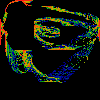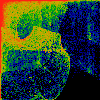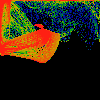# Drips

All of the figures below are constructed with contorted continued fractions. The figures below have not been generated by iterating a function. These figures are not Poincare maps, even though they look like they are. That, in fact is the point.

The full, mathematical depths of the work below is plumbed elsewhere, on the Gap Theory page. But we should take a moment to introduce it anyway. Given a rational r between 0 and 1, it can be expressed as a continued fraction:

r = 1 / (a1 + 1 / (a2 + 1 / (a3 + ...)))

w = 1 / (a1 + z1 / (a2 + z2 / (a3 + ...)))

where the z's are assorted functions of sine and cosine.Csn This shows a scatterplot of (x,y) pairs, with the x's & y's coming from continued fractions, with z of the i'th term being (1+cos(omega*i)) or (1+sin(omega*i)) respectively. All values of omega between 0.0 and 2*pi are equally represented, and account for the cyclic structures. The omega=0.0 values lie roughly on the diagonal.Cycle This shows a single "cycle" of the above, with r ranging from 1/2 to 2/3. Note that all rationals in this range have a one in the first two terms of thier continued fraction expansion. In this, and all of the pictures, the colors represent the "measure": how often a small region (pixel) is visited by the function. Black=never, blue=rarely, green=sometimes, red=often.Far Cycle As above, except that the arguments to sine and cosine are not i*omega, but f(i*omega), where f is the farey number mapping, and omega ranges from 0.0 to 2 Pi.Fnu As above, except that the arguments to sine and cosine are not f(i*omega), but 2*pi*f(i*nu), where f is the farey number mapping, and nu ranges from 0.0 to 1.0.Three Far As above, except that the arguments to sine and cosine are not 2*pi*f(i*nu), but 2*pi*f(3^i *nu), where 3^i is 3 raised to the i'th power. Note that although the derivative of the farey map vanishes at all rationals, it's appearent slope is greatest near 1/3 and 2/3's (and the map is the flatest near 0, 1 and 1/2). The powers-of-three scaling used to generate this figure pairs the most rapidly changing parts of the farey map to the flatest, resulting in the "loops" evident in this figure. Note that the figure appears to almost tile ... but not quite. Note other suggestive horizontal and vertical edges.Far Omega As above, except that the arguments to sine and cosine are not 2*pi*f(3^i *nu), but f(3^i * 2*pi * nu).

# Irates

The "deconvergent"
```c(i) = 1 / (a(i+1) + z(i+1) / (a(i+2) + z(i+2) / (a(i+3) + ...)))
```
gives us yet another quantity to play with.Rat Just as in all of the figures above, this is a scatterplot of cosines vs. sines. This time, though, c(i) is used:

```z(i) = 1.0 + cosine (2*pi*nu*c(i))   for the x coords, and
z(i) = 1.0 +  sine  (2*pi*nu*c(i))   for the y coords.
```Flip Rat As above, but

```z(i) = 1.0 + cosine (2*pi*nu/c(i))   for the x coords, and
z(i) = 1.0 +  sine  (2*pi*nu/c(i))   for the y coords.
```

## Algorithm Notes

Here is a quickie description of the algorithm used to generate the figures above:
```DrawDrips () {
for (int j=0; j < N; j++) {
float r = j/N;
ContunuedFrac c = ComputeContinuedFracOfReal (r);
float x = MangledContinuedFrac (c, cosine);
float y = MangledContinuedFrac (c, sine);

AssignColor (r);
DrawPoint (x,y);
}
}

```
A detailed presentation of the algorithms is presented on the Gap Theory page.
Copyright (c) 1996, 1997 Linas Vepstas